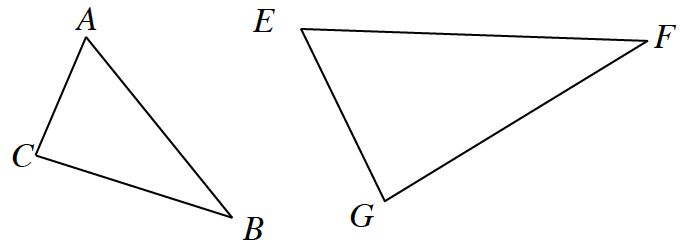### Home > GB8I > Chapter 7 Unit 8 > Lesson INT1: 7.1.2 > Problem7-25

7-25.

On your paper, sketch the two triangles at right and label them with the following information:
$m∠F = 25º$
$m∠A = 73º$
${BC} = {EF} = 44$ cm
$m∠C = m∠G = 82º$

Are the triangles congruent? If so, describe a series of rigid transformations that maps one triangle onto the next. If they are not congruent, explain why not.

Remember that the interior angles of a triangle total 180°.
Set up an equation for each triangle to help find the measure of the unknown angles.
How does this help you to determine if the triangles are congruent?

Remember congruent means that all corresponding angles are equal and all corresponding sides are the same measure.